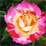# Studio Bluetooth Forum DiscussionsLevel 5Level 5

# pwm issue

Hi  all:

the pwm calculation:

Initial count = 0xFFFF - (Input Clock Freq / PWM Freq out)

Toggle count = 0xFFFF - ((duty cycle in percentage) * (Input Clock Freq / PWM Freq out) / 100)

so the PWM  resolution is 1/100, I want to know if I can change the PWM resolution to 1/1000

Calculation formula:

Initial count = 0xFFFF - (Input Clock Freq / PWM Freq out)

Toggle count = 0xFFFF - ((duty cycle in thousandth) * (Input Clock Freq / PWM Freq out) / 1000)

thanks!

mandy

1 SolutionLevel 5Level 5

# Re: pwm issue